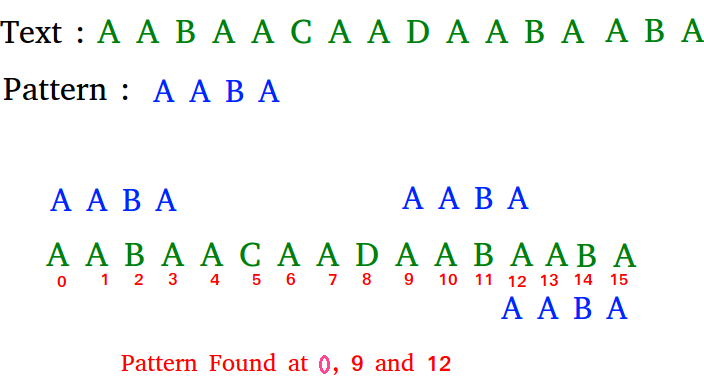# C# Program for Naive algorithm for Pattern Searching

• Last Updated : 11 Dec, 2018

Given a text txt[0..n-1] and a pattern pat[0..m-1], write a function search(char pat[], char txt[]) that prints all occurrences of pat[] in txt[]. You may assume that n > m.

Examples:

```Input:  txt[] = "THIS IS A TEST TEXT"
pat[] = "TEST"
Output: Pattern found at index 10

pat[] =  "AABA"
Output: Pattern found at index 0
Pattern found at index 9
Pattern found at index 12```

Pattern searching is an important problem in computer science. When we do search for a string in notepad/word file or browser or database, pattern searching algorithms are used to show the search results.

## C#

 `// C# program for Naive Pattern Searching ` `using` `System; ` ` `  `class` `GFG { ` ` `  `    ``public` `static` `void` `search(String txt, String pat) ` `    ``{ ` `        ``int` `M = pat.Length; ` `        ``int` `N = txt.Length; ` ` `  `        ``/* A loop to slide pat one by one */` `        ``for` `(``int` `i = 0; i <= N - M; i++) { ` `            ``int` `j; ` ` `  `            ``/* For current index i, check for pattern  ` `            ``match */` `            ``for` `(j = 0; j < M; j++) ` `                ``if` `(txt[i + j] != pat[j]) ` `                    ``break``; ` ` `  `            ``// if pat[0...M-1] = txt[i, i+1, ...i+M-1] ` `            ``if` `(j == M) ` `                ``Console.WriteLine(``"Pattern found at index "` `+ i); ` `        ``} ` `    ``} ` ` `  `    ``// Driver code ` `    ``public` `static` `void` `Main() ` `    ``{ ` `        ``String txt = ``"AABAACAADAABAAABAA"``; ` `        ``String pat = ``"AABA"``; ` `        ``search(txt, pat); ` `    ``} ` `} ` `// This code is Contributed by Sam007 `

Output:

```Pattern found at index 0
Pattern found at index 9
Pattern found at index 13
```

Please refer complete article on Naive algorithm for Pattern Searching for more details!

My Personal Notes arrow_drop_up
Recommended Articles
Page :